# Computing the distance between two integers on a number line Online Quiz

#### Operations Management Online Training

15 Lectures 1 hours

#### Customer Service Excellence - from customers to operations

69 Lectures 6 hours

#### Management Series- Know how to develop a Budget for Business Operations

4 Lectures 33 mins

Following quiz provides Multiple Choice Questions (MCQs) related to Computing the distance between two integers on a number line. You will have to read all the given answers and click over the correct answer. If you are not sure about the answer then you can check the answer using Show Answer button. You can use Next Quiz button to check new set of questions in the quiz.Q 1 - Use the number line to find the distance between the following integers:

−3 and 14

### Explanation

Step 1:

On the number line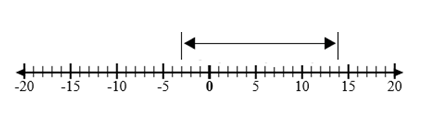Step 2:

Distance between the integers = |14 −(−3) |

|14 + 3 | = |17| = 17 units

Q 2 - Use the number line to find the distance between the following integers:

9 and −6

### Explanation

Step 1:

On the number line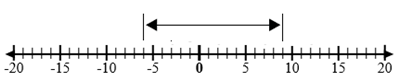Step 2:

Distance between the integers = |9 −(−6) | = 15

Q 3 - Use the number line to find the distance between the following integers:

17 and −8

### Explanation

Step 1:

On the number line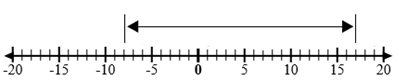Step 2:

Distance between the integers = |17 −(−8) | = 25 units

Q 4 - Use the number line to find the distance between the following integers:

−15 and −6

### Explanation

Step 1:

On the number lineStep 2:

Distance between the integers = |−6 −(−15) | = 9 units

Q 5 - Use the number line to find the distance between the following integers:

18 and 2

### Explanation

Step 1:

On the number line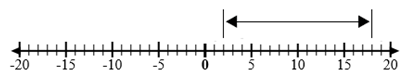Step 2:

Distance between the integers = |18 −(2) | = 16 units

Q 6 - Use the number line to find the distance between the following integers:

12 and 4

### Explanation

Step 1:

On the number line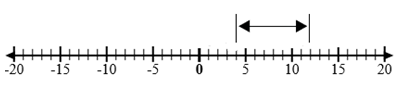Step 2:

Distance between the integers = |12 −(4) | = 8 units

Q 7 - Use the number line to find the distance between the following integers:

6 and 20

### Explanation

Step 1:

On the number line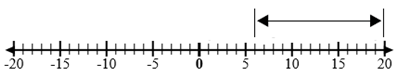Step 2:

Distance between the integers = |20 −(6) | = 14 units

Q 8 - Use the number line to find the distance between the following integers:

−20 and 5

### Explanation

Step 1:

On the number lineStep 2:

Distance between the integers = |5 −(−20) | = 25 units

Q 9 - Use the number line to find the distance between the following integers:

−9 and −3

### Explanation

Step 1:

On the number lineStep 2:

Distance between the integers = |−3 −(−9) | = 6 units

Q 10 - Use the number line to find the distance between the following integers:

−10 and 10

### Explanation

Step 1:

On the number line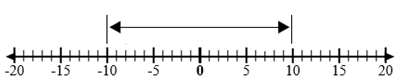Step 2:

Distance between the integers = |10−(−10) | = 20 units

computing_distance_between_two_integers_on_number_line.htm